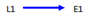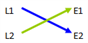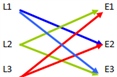# Derangement

Derangement is another important CAT quantitative aptitude topic that holds good weightage and almost every year questions are repeated from this topic. Learn the topic easily here to be able to solve the related questions easily in the exam. Please refer BYJU’S CAT College Predictor tool.

Ultimate Guide to Kickstart your CAT Exam Preparation

### What is Derangement?

Derangement can be simply defined as a permutational arrangement with no fixed points. In other words, derangement can be explained as the permutation of the elements of a certain set in a way that no element of that set appears in their original positions.

The arrangement of 6 people in 6 seats can be done in 6! Ways. Now in how many ways can you arrange them again such that none of them are occupying their original positions?

The question is asking us for the derangement value of 6 people.

Let us consider another case involving the inserting of letters into their respective envelopes.

#### Case 1: Consider one letter L1 and one envelope E1.

In how many ways can the letter be put into a wrong envelope?

Answer will be zero as there is only one letter and one envelope.

There is no way in which you can insert a single letter into the wrong envelope.#### Case 2: Consider two letters L1 and L2 and their two corresponding envelopes E1 and E2.

In how many ways can each letter be put into the wrong envelope?

There will be only one case? when L1 is put into E2, automatically L2 will go into E1Thus, 2 letters can be put in 2 envelopes such that none of them are going into their right envelopes in only one way.

#### Case 3: Take a case now with 3 letters L1, L2, L3 and three envelopes E1, E2, E3.

To find the number of ways in which a letter can be inserted into a wrong envelope will be 2 ways as shown below:

(example- L1 can be put into E2 or E3)3 letters can be put in 3 envelopes such that none of them is going into their right envelopes in 2 ways.

#### Similarly, the derangement of 4 letters will be 9 ways,

5 will be 44, 6 will be 265 and so on..

The calculation of the derangement value of “N” is as follows

DN= N![1-1/1!+1/2!-1/3!…1/N!],

where “N” is the number of items to be de-arranged.

Following are the derangement values of some numbers; it is beneficial to remember these values.

### Illustrations on Derangement:

Question 1: In how many ways can you put 7 letters into their respective envelopes such that exactly 3 go into the right envelope?

a) 24

b) 102

c)315

d) 530

No of ways in which the 3 correct envelopes can be selected= 7C3 =35

Derangement of the remaining 4 envelopes & letters = 9 (derangement value for 4 is 9)

Total No of ways of arrangement = 9 x 35= 315.

Example 2: In how many ways can you form a dancing couple from 3 boys and 3 girls so that no boy dances with his respective girlfriend?

This is clearly a case of derangement of 3 boys and 3 girls.

The value can be calculated as D=3!(1-1/1!+1/2!-1/3!) = 6(2/6) =2 ways.

#### Additional Concept: Sum of all possible numbers and their average formed by “N” digits:

Example 3: Find the sum of all 4 digit numbers which can be formed using the digits 1,2,4 and 5 exactly once.

There is a direct formula to get the answer given by:

Sum of all possible numbers = (n-1)! (sum of the digits involved)(1111…n times)

where n is the number of digits.

Let us find the answer to the above question based on this formula.

Here n= 4;

Thus sum of all possible numbers= (4-1)!(1+2+4+5)(1111)= 3!*(12)*(1111)= 79992.

Suppose you need to find the average of all the possible numbers, you just need to divide it by the total number of possible cases = n!

Average of all possible numbers = (Sum of all possible numbers)/n! = (n-1)!(sum of the digits involved)(1111…n times)/n!

For the above example, the average will be 79992/4! = 3333.

Example 4: What is the average of all five-digit numbers that can be formed using all the digits 1, 2, 3, 4, 5 exactly once?

a) 33453

b) 33333

c) 34333

d) None of these

Solution: Option (b)

To find the average of all numbers, we need to find the sum of all the possible numbers and divide it by the total such numbers possible.

The sum of all possible numbers can be found using the formula (n-1)! (sum of digits)(1111…n times) here n=5.

therefore, (5-1)!(15)(11111)= 4!(15)(11111)

Total number of such possible cases= 5! Average= 3999960/5! = 33333.

Stay tuned with BYJU’S to learn various other CAT exam topics easily and effectively. BYJU’S also provide engaging video lessons and several CAT sample papers to help the candidates prepare better for the CAT exam.